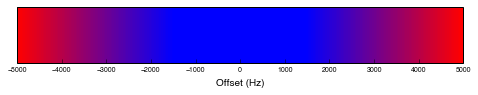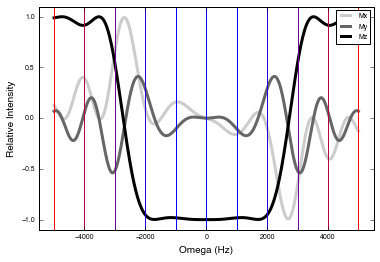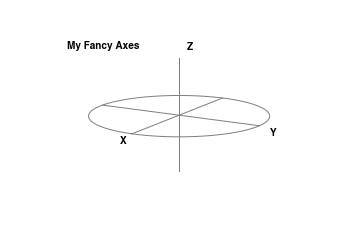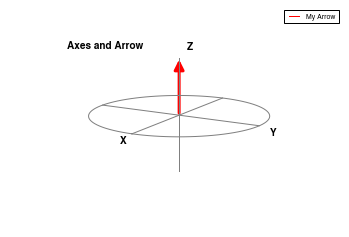# Visualization of NMR Shaped Pulses: Fun with Javascript Animation

This IPython notebook builds on the previous blog post which described how to simulate and plot the result of a shaped pulse on magnetization in an NMR experiment. For the purpose of teaching1, it can also be useful to visualize the effect of this pulse on the magnetization at each discrete time step of the pulse.

Visualizing the time-dependent course of magnetization requires the javascript viewer developed by Jake Vanderplas described here and further demonstrated here. This library has to be installed somewhere in the path searched by Python. NymPy and Matplotlib are also required.

In :
try:
from JSAnimation.IPython_display import display_animation
except:
# clone repo if necessary and load from current directory
! git clone git@github.com:jakevdp/JSAnimation.git tmp
! mv tmp/JSAnimation . && rm -rf tmp

import matplotlib as mpl
from matplotlib.animation import FuncAnimation
%matplotlib inline


The steps required to created the Reburp shaped pulse and calculate its propagator have been described in the aforementioned post and are available in the downloadable and static version of this notebook linked to below. Thus, I have jumped directly to the data visualization.

In :
xyzdata = pulseprop_pulse_steps(relativeomega, pulseShapeInten,
pulseShapePhase, gammaB1max, nu1maxdt,
vectorComponent, n_pulse, n_freq)


The entire frequency range and number of pulse steps are needed for the first graph below, but they will make the animation too large if all are used. So let's reduce them to a smaller set sampled at regular intervals.

In :
freq_skip = 50
pulse_skip = 10
xyzdata_S = xyzdata[:, ::freq_skip, ::pulse_skip]
relativeomega_S = relativeomega[::freq_skip]


## Setup Plot Elements for Animating Vectors in a Spherical Axis¶

A colormap can help visualize a range of data plotted simultaneously in Matplotlib. For the animation, it would be useful to use this colormap as a way to indicate which frequencies are at the limit or outside of the bandwidth of the shaped pulse. This can be accomplished using a custom colormap which is then mapped to the resonance offset range.

In :
from matplotlib.colors import LinearSegmentedColormap, Normalize
from matplotlib.cm import ScalarMappable

def omega_colors(relativeomega):
# First create the colormap where extremes are red, middle is blue
cdict = {'red':   ((0.0,  1.0, 1.0),
(0.35, 0.0, 0.0),
(0.65, 0.0, 0.0),
(1.0,  1.0, 1.0)),
'green': ((0.0, 0.0, 0.0),
(1.0, 0.0, 0.0)),
'blue':  ((0.0,  0.0, 0.0),
(0.35, 1.0, 1.0),
(0.65, 1.0, 1.0),
(1.0,  0.0, 0.0)) }
rbr = LinearSegmentedColormap('RedBlueRed', cdict)

# Normalize the colormap to the extremes of the frequency range
cnorm = Normalize(vmin=np.min(relativeomega), vmax=np.max(relativeomega))

# Generate a blended map over the entire frequency range
# Then get colors for each offset value
scalarMap = ScalarMappable(norm=cnorm, cmap=rbr)
colorList = [scalarMap.to_rgba(x) for x in relativeomega]

return colorList, scalarMap


The colormap can be plotted on its own to see how the colors match up with the frequency range.

In :
colorList, scalarMap = omega_colors(relativeomega)

plt.figure(figsize=(8,1))
ax1 = plt.subplot(111)
cb = mpl.colorbar.ColorbarBase(ax1, cmap=scalarMap.cmap,
norm=scalarMap.norm, orientation='horizontal')
cb.set_label('Offset (Hz)')The colormap can also be used to visualize the frequencies that will be animated on a two-dimensional plot of the net effect of the shaped pulse on the magnetization.

In :
fig = plt.figure()

ax = plt.subplot(111)
ax.plot(relativeomega,xyzdata[0,:,-1],color=(0.8,0.8,0.8,1.0),\
label='Mx',linewidth=3.0)
ax.plot(relativeomega,xyzdata[1,:,-1],color=(0.4,0.4,0.4,1.0),\
label='My',linewidth=3.0)
ax.plot(relativeomega,xyzdata[2,:,-1],color=(0.0,0.0,0.0,1.0),\
label='Mz',linewidth=3.0)
ax.set_xlabel('Omega (Hz)')
ax.set_ylabel('Relative Intensity')
ax.set_xlim(1.1*offset)
ax.set_ylim(-1.1,1.1)
ax.legend()

# Generate the color list using the above function to visualize the frequencies used
# The scalarMap will be used in the animation plot
colorList, scalarMap = omega_colors(relativeomega_S)
for x in range(len(relativeomega_S)):
ax.plot([relativeomega_S[x],relativeomega_S[x]],[-1.1,1.1],color=colorList[x])Matplotlib doesn't have a specific type of three-dimensional axes for plotting vectors on a sphere, so I created a function that will setup one to my liking. Because Matplotlib's text annotation function does not work with three-dimensional axes, a transformation has to be made to calculate the two-dimensional position based on perspective. I am continually amazed by the power of transformations in Matplotlib, and they're worth learning more about.

In :
from mpl_toolkits.mplot3d import Axes3D, proj3d
from mpl_toolkits.mplot3d import proj3d

def circular_axes(fig, title='', elev=20, azim=30):
# Create a 3D figure
ax = fig.gca(projection='3d')

# Draw axes lines
ax.plot([-1,1], [0,0], [0,0], 'gray')
ax.plot([0,0], [-1,1], [0,0], 'gray')
ax.plot([0,0], [0,0], [-1,1], 'gray')

# Draw a gray circle in xy-plane
npoints = 100
ang = np.linspace(0,2*np.pi,npoints)
xcir = np.cos(ang)
ycir = np.sin(ang)
zcir = np.zeros((npoints,))
ax.plot(xcir,ycir,zcir,'gray')
# Uncomment to draw circular axes limits for other planes
#ax.plot(zcir,xcir,ycir,'gray') # Circular permutations!
#ax.plot(ycir,zcir,xcir,'gray')

# Hide the gray background and set perspective
ax.set_axis_off()
ax.view_init(elev,azim)

# Can't use axis_label commands because they will label in the wrong spot
# Annotation commands only work on 2D plots, but can use in 3D plots if
# the 2D projection is calculated
xs_x, ys_x, _ = proj3d.proj_transform(1, 0,0, ax.get_proj())
xs_y, ys_y, _ = proj3d.proj_transform(0, 1,0, ax.get_proj())
xs_z, ys_z, _ = proj3d.proj_transform(0, 0,1, ax.get_proj())
xs_t, ys_t, _ = proj3d.proj_transform(0.5, -1.0, 1, ax.get_proj())

# Label the axes with the calculated 2D points
ax.annotate('X', xy=(xs_x,ys_x), xycoords='data', xytext=(-12,-10),
textcoords='offset points', fontweight='bold', label='xaxis')
ax.annotate('Y', xy=(xs_y,ys_y), xycoords='data', xytext=(10,-10),
textcoords='offset points', fontweight='bold', label='yaxis')
ax.annotate('Z', xy=(xs_z,ys_z), xycoords='data', xytext=(8,8),
textcoords='offset points', fontweight='bold', label='zaxis')
ax.annotate(title, xy=(xs_t,ys_t), xycoords='data', xytext=(-10,8),
textcoords='offset points', fontweight='bold', label='title')

return ax


Let's see what these axes look like.

In :
f = plt.figure()
f.set_facecolor((1.0, 1.0, 1.0, 1.0))
ax = circular_axes(f, 'My Fancy Axes')For vectors plotted on a sphere, I prefer the aesthetic of arrows to simple lines. This is an adaptation of a class written to plot arrows on three-dimensional axes. I added a subroutine for updating the position of an existing arrow as well as a way to create a figure legend for the arrows if desired.

In :
from matplotlib.patches import FancyArrowPatch

class Arrow3D(FancyArrowPatch):
def __init__(self, xs, ys, zs, *args, **kwargs):
FancyArrowPatch.__init__(self, (0,0), (0,0), *args, **kwargs)
self._verts3d = xs, ys, zs
# Probably a cleaner way to save kwargs...
self._label = '_nolegend_'
if 'label' in kwargs.keys():
self._label = kwargs['label']
self._color = 'black'
if 'color' in kwargs.keys():
self._color = kwargs['color']
# Add invisible line for legend
ax = plt.gca() # Prefer to update in 'draw' but it doesn't work
ax.plot3D([0,0], [0,0], [0,0],
linewidth=1.0, label=self._label, color=self._color)

def draw(self, renderer):
xs3d, ys3d, zs3d = self._verts3d
xs, ys, zs = proj3d.proj_transform(xs3d, ys3d, zs3d, renderer.M)
self.set_positions((xs,ys), (xs,ys))
FancyArrowPatch.draw(self, renderer)

# This will update the arrow position
def set_data3d(self, xs3d, ys3d, zs3d):
ax = self.axes
rendererM = ax.get_proj()
xs, ys, zs = proj3d.proj_transform(xs3d, ys3d, zs3d, rendererM)
self._verts3d = xs3d, ys3d, zs3d
self.set_positions((xs, ys), (xs, ys))


Let's see what this looks like with a single arrow drawn.

In :
f = plt.figure()
f.set_facecolor((1.0, 1.0, 1.0, 1.0))
ax = circular_axes(f,'Axes and Arrow')
arrow = Arrow3D([0,0], [0,0], [0,1] ,mutation_scale=20, lw=3.0, \
arrowstyle='-|>', color='red', label='My Arrow')
_ = ax.legend()The last function to declare is for the animation itself--the position of the arrows needs to be updated with each time step. Additionally, it would be nice to be able to rotate the axes at various times. Note that the rotation must be performed before moving the arrows because the position of the arrows is calculated from their projection onto the two-dimensional figure containing the axes.

In :
def update_pos(time, data, arrowList, elevL, azimL):
elev = elevL[time]
azim = azimL[time]
xyz = data[:,:,time]

# Rotate the axis
ax = arrowList.axes
ax.view_init(elev,azim)

# Move the axis labels with the axis
## First get the new projected position
xs_x, ys_x, _ = proj3d.proj_transform(1,0,0, ax.get_proj())
xs_y, ys_y, _ = proj3d.proj_transform(0,1,0, ax.get_proj())
xs_z, ys_z, _ = proj3d.proj_transform(0,0,1, ax.get_proj())
## Find the axis labels
annList = np.array([x for x in ax.get_children() if type(x)==mpl.text.Annotation])
labList = np.array([x.get_label() for x in annList])
xaxLab = annList[np.argwhere(labList=='xaxis')]
yaxLab = annList[np.argwhere(labList=='yaxis')]
zaxLab = annList[np.argwhere(labList=='zaxis')]
## And move them
xaxLab.xy = (xs_x,ys_x)
yaxLab.xy = (xs_y,ys_y)
zaxLab.xy = (xs_z,ys_z)

# Move the arrows
for x in range(len(arrowList)):
arrowList[x].set_data3d([0, xyz[0,x]], [0,xyz[1,x]], [0,xyz[2,x]])


Now we're ready to create the animation.

In :
fig = plt.figure()

# Create the fancy spherical axes
elev = 20
azim = 30
ax = circular_axes(fig,'Reburp Pulse', elev, azim)

# Set the starting position of all the arrows
arrowList = []
for x in range(len(relativeomega_S)):
arrow = Arrow3D([0,0], [0,0], [0,1], mutation_scale=20, lw=2.0,
arrowstyle='-|>', color=colorList[x], label='%.0f Hz'%relativeomega_S[x])
arrowList.append(arrow)

# Option 1: show a colorbar
colorList, scalarMap = omega_colors(relativeomega_S)
scalarMap._A = []
cbar = fig.colorbar(scalarMap, shrink=.6, aspect=10)
cbar.ax.set_yticklabels(['%5.0f Hz'%x for x in relativeomega_S])
# Option 2: show a simple legend
# ax.legend()

# Setup the axis rotation
fpad = 20 # number of frames to pad at the start and finish
mpad = 10 # number of frames to pad in the middle
arotation = np.mod(np.linspace(0, 620., 60) + azim, 360) # azimuthal rotation
apad = len(arotation) # number of frames to pad for azimuthal rotation

# Calculate elevation and azimuthal angles at each time step
azimL = np.append(azimL, arotation)

# Pad the data with starting and finishing values